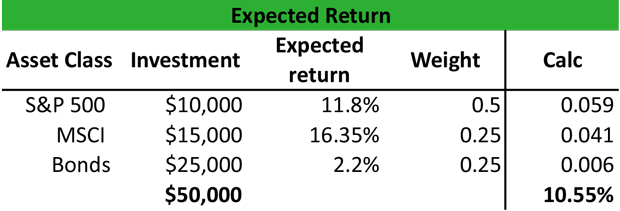# What is an Expected Return?

Definition: Expected returns are profits or losses that investors expect to earn based on anticipated rates of return. Often, the realized returns are different than the expected returns due to the volatility of the markets.

## What Does Expected Return Mean?

What is the definition of expected return? The ER is the profit that an investor anticipates on investment. For instance, it may be the ER on a bond if the bond pays out the maximum return at maturity. In fact, the ER is a possible return on investment, and the outcomes are continuous, i.e. between 0 and infinity.

In portfolio analysis, the returns of a portfolio are the sum of each potential return multiplied by the probability of occurrence or weight. You can also look at it like the ER is the weighted average of potential returns of the portfolio, weighted by the potential returns of each asset class included in the portfolio.

Let’s look at an example.

## Example

Peter holds a portfolio that consists of equity and fixed income with 50% invested in the S&P 500 stock index, 30% invested in the MSCI Emerging Markets index and 20% invested in bonds. The ER on the S&P 500 is 11.8%, for the MSCI is 16.35% and for the bonds is 2.23%. Peter wants to see how this diversification has an impact on his investment strategy and what is the expect return of this portfolio.Peter calculates the portfolio expected return by multiplying the expected return of each asset class to its weight as follows:

Rpf = (11.8% x 0.5) + (16.35% x 0.25) + (2.23% x 0.5) = 0.059 + 0.041 + 0.006 = 10.55%

The return of 10.55% is an assumption that Peter makes based on the weights of each asset class. If the weights change, i.e. the probability changes, then the return will be different. However, the weights used in portfolio analysis are estimated based on the historical performance of each asset class.

## Summary Definition

Define Expected Returns: An expected return is the income or loss from an investment that an investor expects to received based on an assumed rate of return.